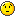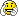# Focusing a TH1I histogram on a previously seen region

Purpose : To focus the display of the histogram on a particular region of interest(ROI)
between repeated calls to load_data. Suppose the histogram has 32000 points
and we want to focus the histogram display between x=1000 and x=2000.
Now suppose we change our ROI to another area using the finger tool widget displayed
when we take the mouse cursor near the x-axis …just below it.
What function to use and where to locate it so that the previously displayed ROI
is maintained between repeated calls to load_data(…)…
Parts of my code is displayed below …
One more help I need is how to label the x-axis depending on the rate of acquisition
of the data points in the histogram.
Suppose the variable rate holds the rate of acquisition of the points
in the 1-D TH1I histogram :
Double_t rate; if rate = 1.0 ; (points acquired at 1 milli second interval)
how to label x-axis in seconds. And if rate = 0.2 ; (0.2 mS) how to label x-axis…
kindly help as the examples given on the internet are not so simple to understand.
forumcpp03.txt (1.86 KB)

Parts of code below :

``````
file1.h
....
public:
TH1I *fHpx_waveMCA;
TAxis* x_waveMCA;
TAxis* y_waveMCA;
Int_t xmaxint_waveMCA;
Int_t xminint_waveMCA;
Double_t xmaxdt;
Double_t xmindt;
Double_t ymaxdt;
Double_t ymindt;
TCanvas *c124;
.....

file1.cpp
...
int Wind1::load_data(unsigned short mod3333, unsigned short chan3333)
{
int retval;
unsigned int NumWords ;
NumWords = 32768;
char hname1 =  "MCA" ;
if (tracehist == NULL)
tracehist = new unsigned int[NumWords];
memset(tracehist, 0, NumWords * sizeof(unsigned int));
printf("Reading Histogram from module: module no:%i , chan no.:%i \n",mod3333, chan3333);
Getdata (tracehist, NumWords, mod3333, chan3333);
if(retval < 0)
{
// error handling
if (retval == -1)
......
return 0;
}
char title01 = "data from module" ;
sprintf(histtitle,"%s,mod=%d,chan=%d", title01 ,mod3333, chan3333);
if (fHpx_waveMCA == NULL ) {
fHpx_waveMCA = new TH1I ( hname1 , histtitle , NumWords, 0, NumWords );
gStyle->SetOptStat(0);
x_waveMCA = fHpx_waveMCA->GetXaxis();
//	y_waveMCA = fHpx_waveMCA->GetYaxis(); // for y-axis
}
fHpx_waveMCA->UseCurrentStyle();
xmaxint_waveMCA = x_waveMCA->GetLast();
xminint_waveMCA = x_waveMCA->GetFirst();
//  for y - axis
//	ymaxdt = fHpx_waveMCA->GetMaximum();
//	ymindt = fHpx_waveMCA->GetMinimum();
fHpx_waveMCA = new TH1I ( hname1 , histtitle , NumWords, 0, NumWords );
fHpx_waveMCA->Reset();
for ( int i = 0; i < NumWords ; i++)
{  fHpx_waveMCA->Fill(i, int(tracehist[i]) );
}
xmaxdt = (Double_t) 2000 ; //   xmaxint_waveMCA;
xmindt = (Double_t) 1000 ; //   xminint_waveMCA;
fHpx_waveMCA->SetAxisRange(xmindt, xmaxdt, "X");
//	fHpx_waveMCA->SetAxisRange(ymindt, ymaxdt, "Y");  // for y-axis
c124->cd();
fHpx_waveMCA->DrawCopy();
c124->Modified();
c124->Update();
gSystem->ProcessEvents();
return 1;
}  // int Wind1::load_data(unsigned short mod3333, unsigned short chan3333)
.....``````

The previous settings I am getting from the following lines before Reset and fill of Histogram :
xmaxint_waveMCA = x_waveMCA->GetLast();
xminint_waveMCA = x_waveMCA->GetFirst();
and I try to set it again after the Reset and fill of the Histogram , but this does not work.
The whole range of the histogram is displayed again…Sorry for the late answer I was away.
Did you find a solution meanwhile ?

No, I have not yet found an answer. I have been thinking about the solution to this problem and
I plan to use two double pointer sliders, one for each axis, placed at the external edges of the
histogram window to set the range of the axis - x and y. when I get time to implement it and get
it working I will post a reply.Ok. Let us know.

I ended up requiring double slider only for the x-axis as the y-axis was auto ranging,
In case I may need it in future then I will have to implement the vertical double
slider also, which is similar to the horizontal double slider for which I have added
the following to my code:

mycode.h

``````#include "TGSlider.h"
#include "TGDoubleSlider.h"
....
public:
TGDoubleHSlider *fHSlider1031;
Float_t	WindowHWmax;
Float_t	WindowHWmin;
....

In mycode.cpp file in constructor:
....
fHSlider1031 = new TGDoubleHSlider(fVerticalFrame770,1016, kScaleBoth ,-1,kHorizontalFrame);
fHSlider1031->SetName("fHSlider1031");
fHSlider1031->SetRange(0,int (NumWords) );  // NumWords holds the max range value of x-axis
fHSlider1031->SetPosition(0,9000);
fHSlider1031->Associate(this);
fHSlider1031->MoveResize(0,544,1016,24);
....
Bool_t HistWin::ProcessMessage (Long_t msg, Long_t parm1, Long_t parm2)
{
cout << "msg = " << msg << ", parm1 = " << parm1 << ", parm2 = " << parm2 << '\n' ;
switch (GET_MSG (msg))
{
case kC_COMMAND:
.....
break;
......
case kC_HSLIDER:
{	cout << "I am in Slider code" << "\n" ;
WindowHWmax = fHSlider1031->GetMaxPosition();
WindowHWmin = fHSlider1031->GetMinPosition();
cout << "WindowHWmin = " << WindowHWmin << " WindowHWmax = " << WindowHWmax "\n";
fHpx_waveMCA->SetAxisRange(WindowHWmin, WindowHWmax, "X");
cout << "I am here 007" << "\n";
c124->cd();cout << "I am here 008" << "\n";
fHpx_waveMCA->DrawCopy();cout << "I am here 009" << "\n";
c124->Modified();cout << "I am here 010" << "\n";
c124->Update();cout << "I am here 011" << "\n";
gSystem->ProcessEvents();cout << "I am here 012" << "\n";
}
break;
default:
break;
....

The code works excellently, brilliantly, simply superb,…